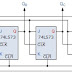In the previous tutorial we saw that an Asynchronous counter can have 2n-1 possible counting states e.g. MOD-16 for a 4-bit counter, (0-15) making it ideal for use in Frequency Division. But it is also possible to use the basic asynchronous counter to construct special counters with counting states less than their maximum output number.
This is achieved by forcing the counter to reset itself to zero at a pre-determined value producing a type of asynchronous counter that has truncated sequences. Then an n-bit counter that counts up to its maximum modulus ( 2n ) is called a full sequence counter and a n-bit counter whose modulus is less than the maximum possible is called a truncated counter.
But why would we want to create an asynchronous truncated counter that is not a MOD-4, MOD-8, or some other modulus that is equal to the power of two. The answer is that we can by using combinational logic to take advantage of the asynchronous inputs on the flip-flop. If we take the modulo-16 asynchronous counter and modified it with additional logic gates it can be made to give a decade (divide-by-10) counter output for use in standard decimal counting and arithmetic circuits.
Such counters are generally referred to as Decade Counters. A decade counter requires resetting to zero when the output count reaches the decimal value of 10, ie. when DCBA = 1010 and to do this we need to feed this condition back to the reset input. A counter with a count sequence from binary “0000” (BCD = “0”) through to “1001” (BCD = “9”) is generally referred to as a BCD binary-coded-decimal counter because its ten state sequence is that of a BCD code but binary decade counters are more common.

This type of asynchronous counter counts upwards on each trailing edge of the input clock signal starting from 0000 until it reaches an output 1001 (decimal 9). Both outputs QA and QD are now equal to logic “1”. One the application of the next clock pulse, the output from the 74LS10 NAND gate changes state from logic “1” to a logic “0” level.
As the output of the NAND gate is connected to the CLEAR CLR ) inputs of all the 74LS73 J-K Flip-flops, this signal causes all of the Q outputs to be reset back to binary 0000 on the count of 10. As outputs QA and QD are now both equal to logic “0” as the flip-flop’s have just been reset, the output of the NAND gate returns back to a logic level “1” and the counter restarts again from 0000. We now have a decade or Modulo-10 up-counter.
Asynchronous Counter - By B.SURESH ,Department of ECE,Reviewed by Suresh Bojja on 9/07/2015 09:40:00 AM Rating: 5